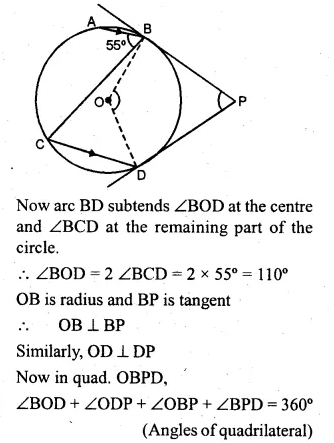Guru

# Question 24. (a) In the figure (i) given below, O is the centre of the circle. The tangent at B and D meet at P. If AB is parallel to CD and ∠ ABC = 55°. find: (i)∠BOD (ii) ∠BPD (b) In the figure (ii) given below. O is the centre of the circle. AB is a diameter, TPT’ is a tangent to the circle at P. If ∠BPT’ = 30°, calculate : (i)∠APT (ii) ∠B OP.

• 1

This is circle based question from Chapter name- circles
Topic – Angle properties of circles
Chapter number- 15

In the question , O is the centre of the circle. The tangent at B and D meet at P.

If AB is parallel to CD and ∠ ABC = 55°.

Now we have to find: (i)∠BOD (ii) ∠BPD

b) in the given figure O is the centre of the circle. AB is a diameter,

TPT’ is a tangent to the circle at P. If ∠BPT’ = 30°,

Now we have to calculate : (i)∠APT (ii) ∠B OP.

ICSE Avichal publication
Understanding ICSE Mathematics
Question no 24

Share